# How to Compare and Order Decimals

How to Compare and Order Decimals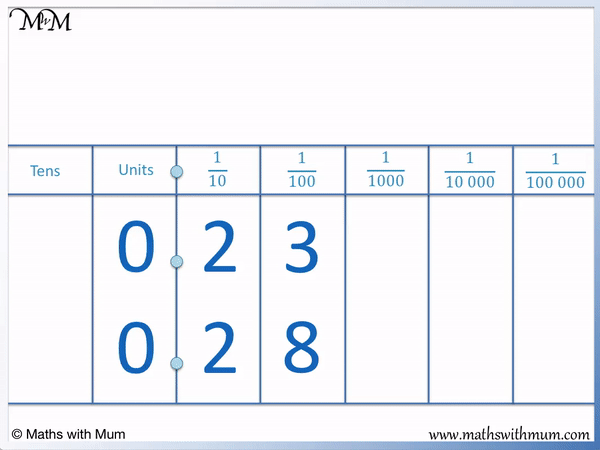• To compare two decimals, first line up the decimal points and digits in each place value column directly above each other.
• We compare the individual digits in each place value column from left to right.
• In the ones column, both digits are 0 and so these digits are equal.
• We move on to compare the next place value column to the right.
• Both digits are 2 and so they are also equal.
• We move on to compare the next place value column to the right.
• 8 is greater than 3 and so, the decimal with the 8 is the largest.
• 0.28 is larger than 0.23.
Line up the digits in each place value column and compare them individually from left to right.

The first digit that is largest indicates the largest decimal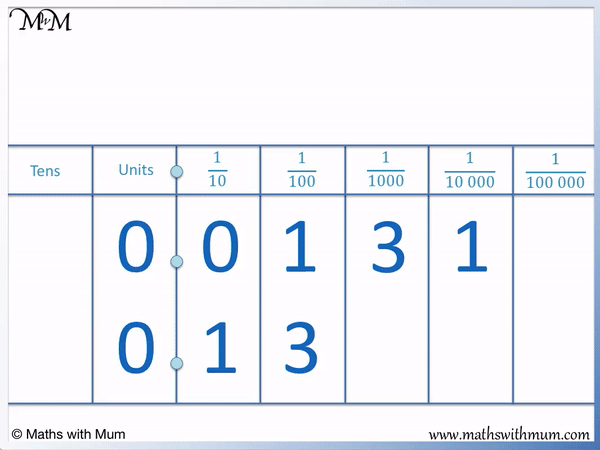• We first line up the decimal points and the place value columns of each number directly above each other.
• We compare the digits in each column individually from left to right.
• Both numbers have a 0 in the ones column so we move on to the next column.
• In the tenths column, 1 is larger than 0.
• Therefore 0.13 is a larger decimal than 0.0131.
• We do not need to compare any more digits. We stop comparing as soon as one digit of one number is larger than the other.
• Even though 0.0131 has more digits, it is a smaller number than 0.13 because it has 0 tenths.Supporting Lessons# Ordering and Comparing Decimals

## How to Compare Decimals

To compare decimals, use the following steps:

1. Line up the digits in each place value column directly above another.
2. Compare the digits in each place value column individually from left to right.
3. If the digits are equal, compare the digits in the next column to the right.
4. If a digit is larger than the others, this digit belongs to the largest decimal.

For example, we will compare the two decimals 0.1 and 0.3.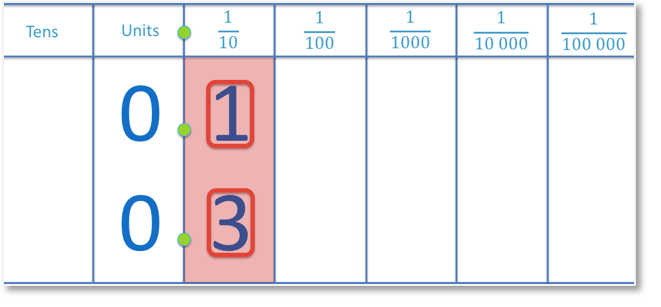The first step is to line up the digits in each place value column. When teaching this, it is useful to start by lining up the decimal points and then writing each digit directly above the others. Square grid paper can be useful for ensuring that the digits are written above another.

The second step is to look at the digits in the place value columns, starting on the left and moving right.

The left-most digits are in the ones column and they are both 0. They are equal.

If the digits are equal, we compare the digits in the next place value column to the right.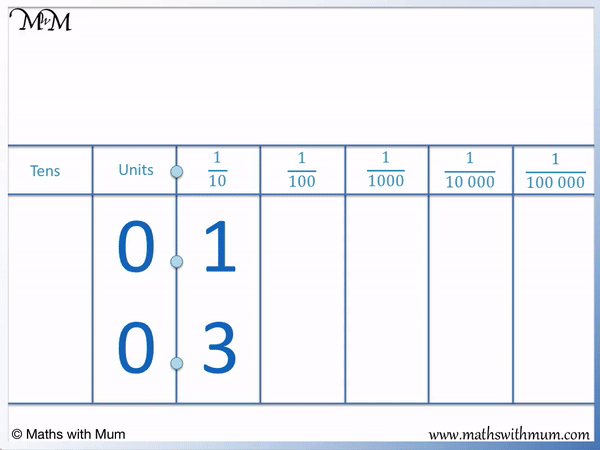3 is greater than 1 and the 3 belongs to the decimal 0.3. Therefore 0.3 is a larger decimal than 0.1.

The two numbers do not have a whole number component as there are 0 ones in both numbers. 0.3 has 3 tenths, whereas 0.1 only has 1 tenth.

We can compare two decimals using a decimal model.In this model, the whole square represents one whole unit and each column is one tenth.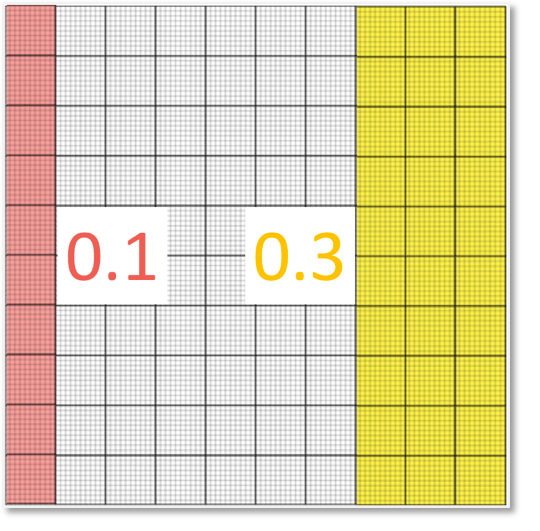We can see that 0.1, shown in red, is one tenth and 0.3, shown in yellow, has three tenths. 0.3 is clearly larger than 0.1.

Here is an example of comparing the decimals 0.2 and 0.14.

We first line the digits up above one another.Again, the ones digit is 0 in both numbers. We move on to compare the digits in the tenths column.

2 is larger than 1 and so stop. 0.2 is larger than 0.14.As soon as one digit is larger than another digit in the same place value column, this belongs to the larger decimal. There is no need to compare any other digits in the columns to the right.

A common misconception is that the more digits in the decimal, the bigger the number. This is not a rule and it will often result in the wrong answer.

In this example, some people might think that 0.14 is larger than 0.2 because 14 is bigger than 2. We cannot compare decimal numbers by simply reading the decimal numbers as though they were whole numbers.

0.2 is larger than 0.14 because 0.2 contains 2 tenths, whereas 0.14 contains only 1 tenth.

Here is a decimal model to show the comparison in size of these decimals.We can clearly see that 0.2 is a larger decimal than 0.14.

We say that 0.2 is greater than 0.14 and this can be written mathematically as 0.2 > 0. 14.Here is another example of comparing two decimal numbers. We have 0.3215 and 0.3211.

We write the digits directly above each other and compare them from left to right.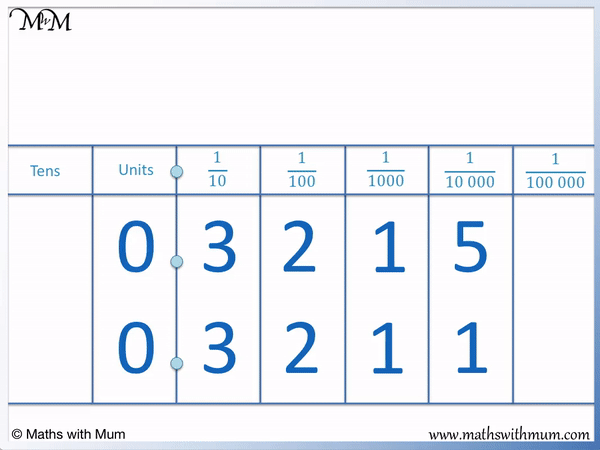The digits are the same in the ones column, they are both 0.

The digits are the same in the tenths column, they are both 3.

The digits are the same in the hundredths column, they are both 2.

The digits are the same in the thousandths column, they are both 1.

Finally, in the ten-thousandths column, 5 is larger than 1.

Therefore 0.3215 is larger than 0.3211. It is larger by only 4 ten-thousandths, which is by a very small amount.

The two decimals are shown in a model below.## Comparing Decimals with Models

To compare decimals with models, use base ten blocks to represent each place value. Collect the number of tenths, hundredths and thousandths together and see which amount is the largest.

Here is a visual image for modelling tenths, hundredths and thousandths.The entire square shown is worth one whole unit. You can use a base ten block flat to represent one whole if teaching this to a child.

Each column rod is worth one tenth because ten of these rods make the whole square.

Each individual unit cube can be worth one hundredth.

For smaller quantities, you may wish to draw the diagrams as they are shown on this page.Here the two rods in 0.23 and 0.28 are both shown by the two complete vertical columns.

0.23 has 3 more hundredths, which are shown by the three squares.

0.28 has 8 more hundredths, shown by the 8 squares.

When teaching comparing decimals, is useful to introduce decimals using decimal models before then proceeding onto comparing decimals digit by digit. Eventually, once you have grasped the relative size of decimals, it is not necessary to keep drawing out models each time.Now try our lesson on Rounding Decimals to the Nearest Whole Number where we learn how to write a decimal to its nearest whole number.